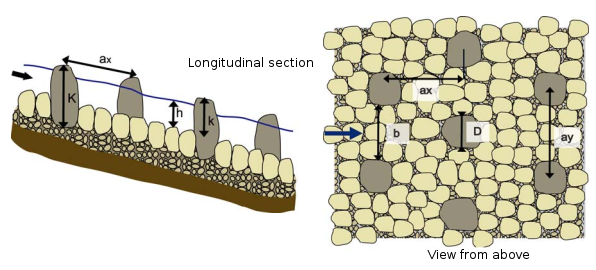# Blocks concentration

This module makes it possible to calculate the concentration of uniformly distributed blocks in a rock-ramp fish pass.

It allows to calculate one of the following values:

• The width of the pass (m) ;
• The number of blocks ;
• The diameter of the blocks (m) ;
• The concentration of the blocks $$C$$.

## FormulaExcerpt from Larinier et al., 20061

The spacing between the blocks is then calculated with the following formula:

$ax = ay = \frac{D}{\sqrt{C}}$

With :

• $$ax$$ and $$ay$$ respectively the longitudinal and lateral distances between the centres of the blocks (m);
• the width of the blocks facing the flow (m);
• the concentration of the blocks (-).

The number of blocks $$N$$ for a pass of width $$B$$ is then obtained with the equation:

$N = B / ax$

## Harmonization

When calculating the number of blocks, if it is not an integer, upward and downward harmonized numbers of blocks are given along with corresponding concentration values.

Tolerance is of the order of a centimetre.

1. Larinier, Michel, Courret, D., Gomes, P., 2006. Technical guide for the design of "natural" fish passes, GHAPPE RA Report. Compagnie Nationale du Rhône / Adour Garonne Water Agency. http://dx.doi.org/10.13140/RG.2.1.1834.8562